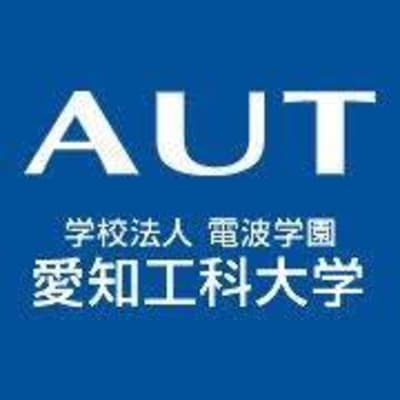# 愛知工科大学## Introduction

Mathematical exploration power:
Students are required to take and acquire a group of mathematical subjects in high school with the ability to find out the characteristics of the problem, use the relevant mathematical knowledge and logical ability, and solve the problem.